# Texas Go Math Grade 5 Lesson 13.1 Answer Key Appropriate Tools and Units

Refer to our Texas Go Math Grade 5 Answer Key Pdf to score good marks in the exams. Test yourself by practicing the problems from Texas Go Math Grade 5 Lesson 13.1 Answer Key Appropriate Tools and Units.

## Texas Go Math Grade 5 Lesson 13.1 Answer Key Appropriate Tools and Units

Unlock the Problem

Mr. Lee wants to find Eric’s height. What tool and unit should Mr. Lee use to measure Eric’s height?

Tell which units are measured by each tool.So, Mr. Lee would use a “Yardstick” to measure Eric’s height, and the unit he would use is “yard”.

Tell which units are measured by each tool.Math Talk
Mathematical processes
Explain how a farmer might weigh sheep for the fair?
A farmer might weigh sheep for the fair in terms of “Pounds”

Example

Mr. Wilkins takes his dog to the vet. What tool and unit would the vet use to measure the dog’s mass?Some metric units for measuring mass are grams, kilograms, and milligrams.
Some tools that can be used are a pan balance and a large scale.
So, the vet would use a _”Large Scale” to measure the dog’s mass, and the unit he would use is “Kilograms”Try This! Tell which units are measured by each tool.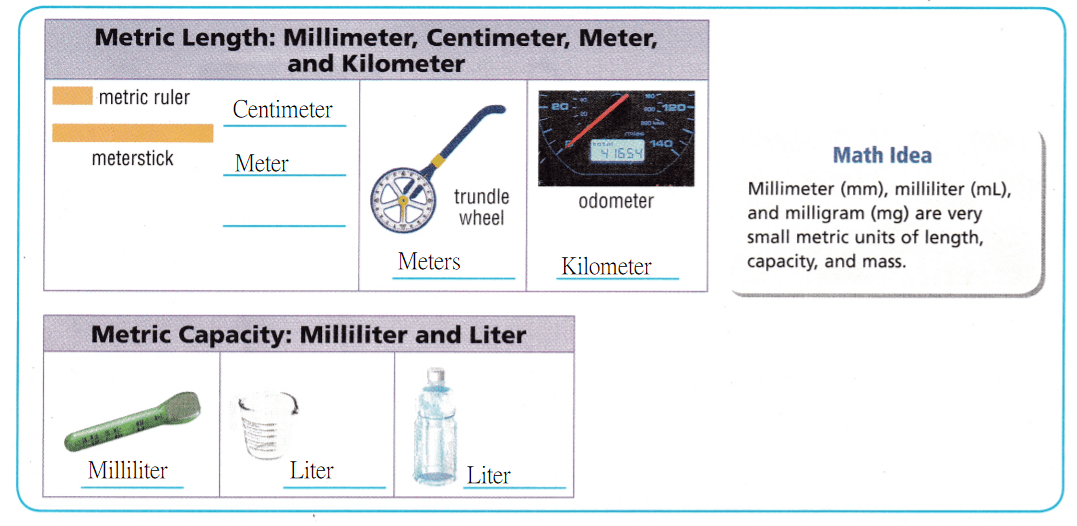Share and Show
Choose the appropriate metric tool and unit.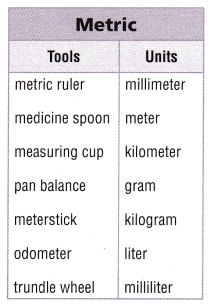Question 1.
length of a pencil
____ _____
The given table is:Now,
From the given table,
We can observe that
The metric tool used to measure the length of the pencil is: Metric ruler
The unit used to measure the length of the pencil is: Millimeter
Hence, from the above,
We can conclude that
The metric tool used to measure the length of the pencil is: Metric ruler
The unit used to measure the length of the pencil is: Millimeter

Question 2.
mass of a strawberry
___ _____
The given table is:Now,
From the given table,
We can observe that
The metric tool used to measure the mass of a strawberry is: Pan balance
The unit used to measure the mass of a strawberry is: Gram
Hence, from the above,
We can conclude that
The metric tool used to measure the mass of a strawberry is: Pan balance
The unit used to measure the mass of a strawberry is: Gram

Question 3.
The capacity of a medicine bottle
____ _____
The given table is:Now,
From the given table,
We can observe that
The metric tool used to measure the capacity of a medicine bottle is: Trundle wheel
The unit used to measure the capacity of a medicine bottle is: Millimeter
Hence, from the above,
We can conclude that
The metric tool used to measure the capacity of a medicine bottle is: Trundle wheel
The unit used to measure the capacity of a medicine bottle is: Millimeter

Problem Solving

Question 4.
H.O.T. Multi-Step Maria used one customary unit to measure the height of a tomato plant when it was first planted and a different customary unit when it was fully grown. She used the same measurement tool both times. What tool did she use? What unit did she use each time?
It is given that
Maria used one customary unit to measure the height of a tomato plant when it was first planted and a different customary unit when it was fully grown. She used the same measurement tool both times
Now,
According to the given information,
The measurement tool used to measure the height of a tomato plant when it was first planted and when it was fully grown is: Metric scale
The unit used to measure the height of a tomato plant when it was first planted is: Centimeters
The unit used to measure the height of a tomato plant when it was fully grown is: Meters
Hence, from the above,
We can conclude that
The measurement tool used to measure the height of a tomato plant when it was first planted and when it was fully grown is: Metric scale
The unit used to measure the height of a tomato plant when it was first planted is: Centimeters
The unit used to measure the height of a tomato plant when it was fully grown is: Meters

Question 5.
Jill wants to know the length of a toy car in metric units. What tool and unit will she use?
It is given that
Jill wants to know the length of a toy car in metric units.
Now,
According to the given information,
The measurement tool used to know the length of a toy car is: Measuring tape
The unit that is used to know the length of a toy car is: Centimeters
Hence, from the above,
We can conclude that
The measurement tool used to know the length of a toy car is: Measuring tape
The unit that is used to know the length of a toy car is: Centimeters

Question 6.
Use Tools Glen used ounces to measure the weight of an object. What tool did he use? What could the object be?
It is given that
Glen used ounces to measure the weight of an object
Now,
According to the given information,
The tool did Glen used to measure the weight of an object is: Scale (or) Balance
The objects that use the unit of “Ounces” are A slice of whole-grain bread, a CD, etc.
Hence, from the above,
We can conclude that
The tool did Glen used to measure the weight of an object is: Scale (or) Balance
The objects that use the unit of “Ounces” are A slice of whole-grain bread, a CD, etc.

Problem Solving

Question 7.
H.O.T. Reasoning Would an inch ruler be a reasonable tool to use to measure the height of a water tower that is more than 68 feet tall? Explain.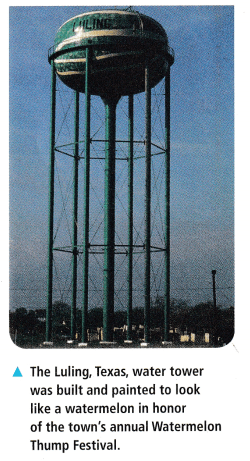It is given that
The height of a water tower is more than 68 feet tall
Now,
We know that,
The length of inch scale should be less than the feet scale
Now,
According to the given information,
An inch ruler should not be a reasonable tool to use to measure the height of a water tower that is more than 68 feet tall
Hence, from the above,
We can conclude that
An inch ruler should not be a reasonable tool to use to measure the height of a water tower that is more than 68 feet tall

Question 8.
Explain Which customary unit would most likely be used to measure the capacity of a water tower? Why?
We know that,
The water tower occupies the large space
So,
The customary unit that would most likely be used to measure the capacity of a watchtower is: Liters
Hence, from the above,
We can conclude that
The customary unit that would most likely be used to measure the capacity of a watchtower is: Liters

Question 9.
Multi-Step What tool would you use to find the perimeter of a rectangular patio? What unit would you use?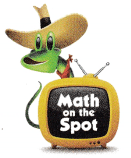We know that,
The size of a rectangular patio is generally in terms of feet
So,
The unit that is used to measure the rectangular patio is: Measuring tape
Hence, from the above,
We can conclude that
The unit that is used to measure the rectangular patio is: Measuring tape

Question 10.
Pablo swims 1 length of a swimming pool. What unit would be best for measuring the length of the pool?
(A) inch
(B) centimeter
(C) foot
(D) kilometer
It is given that
Pablo swims 1 length of a swimming pool
Hence, from the above,
We can conclude that
The unit that would be best for measuring the length of the pool is:Question 11.
Which of the following would you most likely measure in grams?
(A) mass of a berry
(B) mass of a watermelon
(C) length of a berry
(D) height of a watermelon
The following that is used to measure most likely in grams is: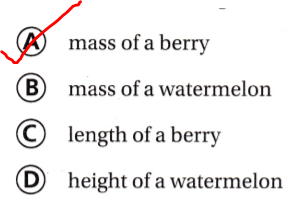Question 12.
Multi-Step Mia used a measuring cup to find a particular measurement. She recorded the measurement as 5 liters. What could Mail have been measuring?
(A) weight of a punch bowl
(B) tThe capacity of a punch bowl
(C) weight of a cereal bowl
(D) The capacity of a cereal bowl
It is given that
Mia used a measuring cup to find a particular measurement. She recorded the measurement as 5 liters
Hence, from the above,
We can conclude that
The object mail could have been measuring is:Texas Test Prep

Question 13.
Use Tools Which tool would you use to measure the capacity of a coffee mug?
(A) meterstick
(B) spring scale
(C) measuring cup
(D) 1-liter container
The tool that is used to measure the capacity of a coffee mug is:### Texas Go Math Grade 5 Lesson 13.1 Homework and Practice Answer Key

Choose the appropriate metric tool and unit.Question 1.
height of a soccer trophy
___ _____
The given table is:Now,
From the given table,
We can observe that
The metric tool used to measure the height of a soccer trophy is: Metric ruler
The unit used to measure the length of the pencil is: Centimeter
Hence, from the above,
We can conclude that
The metric tool used to measure the height of a soccer trophy is: Metric ruler
The unit used to measure the length of the pencil is: Centimeter

Question 2.
driving distance from home to the soccer field
_____ _____
The given table is:Now,
From the given table,
We can observe that
The metric tool used to measure the driving distance from home to the soccer field is: Odometer
The unit used to measure the driving distance from home to the soccer field is: Kilometer
Hence, from the above,
We can conclude that
The metric tool used to measure the driving distance from home to the soccer field is: Odometer
The unit used to measure the driving distance from home to the soccer field is: Kilometer

Question 3.
length of a soccer field _____
The given table is:Now,
From the given table,
We can observe that
The metric tool used to measure the length of a soccer field is: Metric ruler
The unit used to measure the length of a soccer field is: Kilometer
Hence, from the above,
We can conclude that
The metric tool used to measure the length of a soccer field is: Metric ruler
The unit used to measure the length of a soccer field is: Kilometer

Question 4.
The capacity of a bucket
The given table is:Now,
From the given table,
We can observe that
The metric tool used to measure the capacity of a bucket is: Measuring cup
The unit used to measure the capacity of a bucket is: Liter
Hence, from the above,
We can conclude that
The metric tool used to measure the capacity of a bucket is: Measuring cup
The unit used to measure the capacity of a bucket is: Liter

Question 5.
mass of a coin
The given table is:Now,
From the given table,
We can observe that
The metric tool used to measure the mass of a coin is: Pan balance
The unit used to measure the mass of a coin is: Gram
Hence, from the above,
We can conclude that
The metric tool used to measure the mass of a coin is: Pan balance
The unit used to measure the mass of a coin is: Gram

Question 6.
length of a car
The given table is:Now,
From the given table,
We can observe that
The metric tool used to measure the length of a car is: Meterstick
The unit used to measure the length of a car is: Meter
Hence, from the above,
We can conclude that
The metric tool used to measure the length of a car is: Meterstick
The unit used to measure the length of a car is: Meter

Question 7.
The capacity of an eyedropper
The given table is:Now,
From the given table,
We can observe that
The metric tool used to measure the capacity of an eyedropper is: Trundle wheel
The unit used to measure the capacity of an eyedropper is: Milliliter
Hence, from the above,
We can conclude that
The metric tool used to measure the capacity of an eyedropper is: Trundle wheel
The unit used to measure the capacity of an eyedropper is: Milliliter

Question 8.
mass of an orange
The given table is:Now,
From the given table,
We can observe that
The metric tool used to measure the mass of orange is: Pan balance
The unit used to measure the mass of orange is: Gram
Hence, from the above,
We can conclude that
The metric tool used to measure the mass of orange is: Pan balance
The unit used to measure the mass of orange is: Gram

Question 9.
length of a school building
The given table is:Now,
From the given table,
We can observe that
The metric tool used to measure the length of a school is: Meterstick
The unit used to measure the length of a school is: Kilometer
Hence, from the above,
We can conclude that
The metric tool used to measure the length of a school is: Meterstick
The unit used to measure the length of a school is: Kilometer

Problem Solving

Question 10.
The Guadalupe River is a popular destination for rafters and canoers. Which customary unit would most likely be used to measure the length of a river? Why?
It is given that
The Guadalupe River is a popular destination for rafters and canoers
So,
The customary unit that would most likely be used to measure the length of a river is: Kilometer
We use “Kilometer” to measure the long distances
Hence, from the above,
We can conclude that
The customary unit that would most likely be used to measure the length of a river is: Kilometer
We use “Kilometer” to measure the long distances

Question 11.
Would a yardstick be a reasonable tool to use to measure the length of a canoe paddle? Explain
We know that,
The length of a canoe paddle is generally 52 inches to 62 inches
So,
The “Inchstick” is reasonable to measure the length of a canoe paddle
Hence, from the above,
We can conclude that
A “Yardstick” should not be a reasonable tool to measure the length of a canoe paddle

Texas Test Prep

Lesson Check

Question 12.
Which of the following would you most likely measure in kilograms?
(A) mass of a paper clip
(B) length of a horseshoe
(C) length of a longhorn cow
(D) mass of a longhorn cow
The following that you would most likely measure in Kilograms is: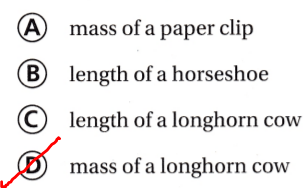Question 13.
Which tool would you use to measure the mass of a mobile phone?
(A) meterstick
(B) trundle wheel
(C) pan balance
(D) odometer
The tool that is used to measure the mass of a mobile phone is: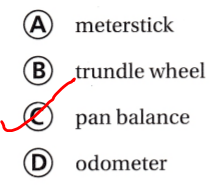Question 14.
Every spring, cyclists participate in a two-day fundraising ride from Houston to Austin. Which unit would be best for measuring the distance they ride?
(A) meter
(B) kilometer
(C) foot
(D) yard
It is given that
Every spring, cyclists participate in a two-day fundraising ride from Houston to Austin
Hence, from the above,
We can conclude that
The unit that would be best for measuring the distance the cyclists ride is: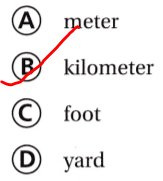Question 15.
Kaylee measures honey into a bowl with ingredients for a batch of muffins. Which is the most likely measurement for the honey?
(A) 3 tablespoons
(B) 3 gallons
(C) 3 liters
(D) 3 kilograms
It is given that
Kaylee measures honey into a bowl with ingredients for a batch of muffins
Hence, from the above,
We can conclude that
The unit that is most likely to be the measurement for the honey is:Question 16.
Brian serves ice cream to his family. Which is the most likely measurement for the capacity of the ice cream carton?
(A) 1 cup
(B) 1 gallon
(C) 1 teaspoon
(D) 1 millimeter
It is given that
Brian serves ice cream to his family
Hence, from the above,
We can conclude that
The unit that is most likely to be the measurement for the capacity of the ice cream carton is: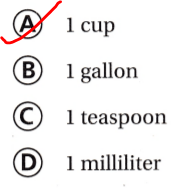Question 17.
Which container would most likely have a capacity that is measured in liters?
(A) a drinking glass
(B) a watering can
(C) a baby bottle
(D) a soup spoon
The container that would most likely have a capacity that is measured in liters is: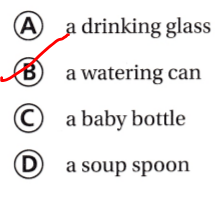Question 18.
Multi-Step Tristan used a measuring cup to find a particular measurement. He recorded the measurement as 6 cups. What could Tristan have been measuring?
(A) The capacity of a mixing howl
(B) weight of a mixing bowl
(C) The capacity of a juice glass
(D) weight of a juice glass
It is given that
Tristan used a measuring cup to find a particular measurement. He recorded the measurement as 6 cups
Hence, from the above,
We can conclude that
The object that could Tristan have been measuring is: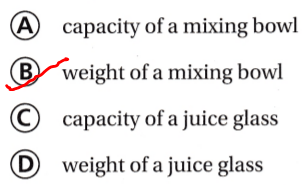Question 19.
Multi-Step A scientist measured a museum specimen. She recorded the measurement as 75 centimeters. What could the scientist have been measuring?
(A) length of a dinosaur bone
(B) mass of a dinosaur hone
(C) length of a gem
(D) mass of a gem